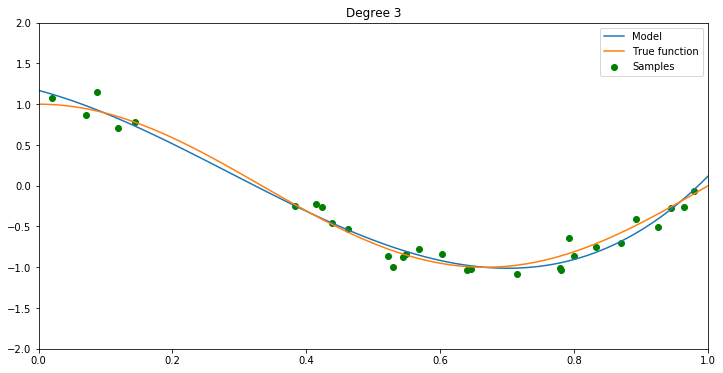# MLF - Overfitting and Underfitting

Last updated: July 22nd, 2020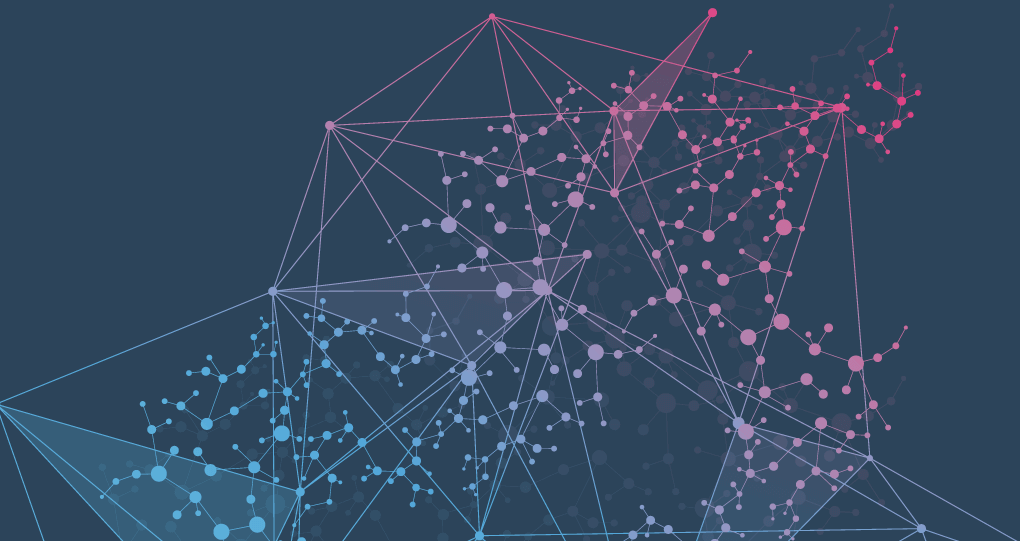# Overfitting and underfitting¶

The cause of poor performance in machine learning is either overfitting or underfitting the data.

In this lesson, we will learn what these concepts mean and the problems they lead to.## Target function¶

Supervised machine learning is best understood as approximating a target function ($f$) that maps input variables ($X$) to an output variable ($y$).

$$y = f(X)$$

This characterization describes the range of classification and prediction problems and the machine algorithms that can be used to address them.

An important consideration in learning is that the target function from the training data is how well the model generalizes to new data. Generalization is important because the data we collect is only a sample, it is incomplete and noisy.

### Generalization¶

In supervised learning, we want to build a model on the training data and then be able to make accurate predictions on new, unseen data that has the same characteristics as the training set that we used.

If a model is able to make accurate predictions on unseen data, we say it is able to generalize from the training set we trained it. We want to build a model that is able to generalize as accurately as possible on new data.

### Signal vs. Noise¶

In predictive modeling, you can think of the signal as the true underlying pattern that you wish to learn from the data.

Noise on the other hand, refers to the irrelevant information or randomness in a dataset.## The Bias-Variance trade-off¶

Bias and variance are two terms you need to get used to if constructing statistical models, such as those in machine learning.

• Bias is the difference between your model's expected predictions and the true values.
• Variance refers to your algorithm's sensitivity to specific sets of training data.

There is a tension between wanting to construct a model which is complex enough to capture the system that we are modelling, but not so complex that we start to fit to noise in the training data. This is related to underfitting and overfitting of a model to data, and back to the bias-variance tradeoff.

This trade-off between too simple (high bias) vs. too complex (high variance) is a key concept in statistics and machine learning, and one that affects all supervised learning algorithms.

• Increasing the bias will decrease the variance.
• Increasing the variance will decrease the bias.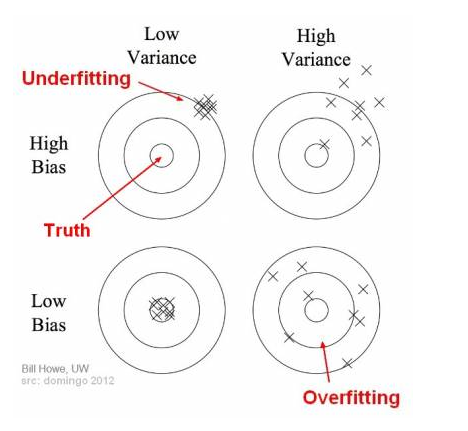• Low Bias: Suggests less assumptions about the form of the target function.
• High Bias: Suggests more assumptions about the form of the target function.
• Low Variance: Suggests small changes to the estimate of the target function with changes to the training dataset.
• High Variance: Suggests large changes to the estimate of the target function with changes to the training dataset.

Low variance (high bias) algorithms tend to be less complex, with simple or rigid underlying structure. Linear machine learning algorithms often have a high bias but a low variance.

On the other hand, low bias (high variance) algorithms tend to be more complex, with flexible underlying structure. Nonlinear machine learning algorithms often have a low bias but a high variance.## Overfitting, underfitting and "good fit"¶

Getting the right complexity of a model is one of the key skills in developing any kind of statistically based model.

Overfitting refers to a model that models the training data too well, performing well on the training dataset but does not perform well on a hold out sample. This happens because the model has learned the noise instead of the signal -good data-.

Underfitting refers to a model that can neither model the training data nor generalize to new data. This model fails to sufficiently learn the problem and performs poorly on a training dataset and does not perform well on a holdout sample.

Good Fit. A model that suitably learns the training dataset and generalizes well to the old out dataset. This is the goal, but is very difficult to do in practice.

In statistics, goodness of fit refers to how closely a model's predicted values match the observed (true) values.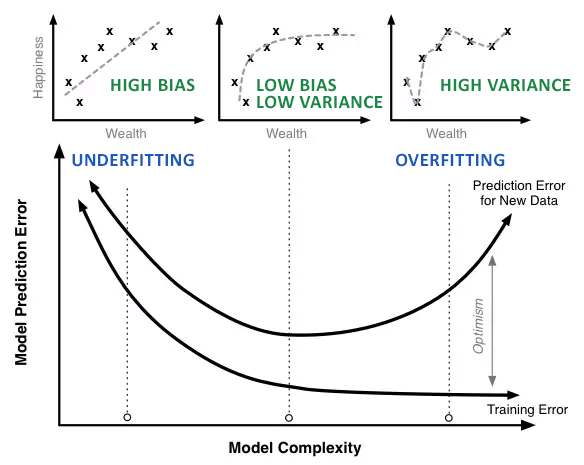A model that generalizes well is a model that is neither underfit nor overfit.

### How can we detect overfitting?¶

A key challenge with overfitting, and with machine learning in general, is that we can't know how well our model will perform on new data until we actually test it.

If our model does much better on the training set than on the test set, then we're likely overfitting.

Another tip is to start with a very simple model to serve as a benchmark. Then, as you try more complex algorithms, you'll have a reference point to see if the additional complexity is worth it.

### Ways to prevent overfitting¶

There are a few ways prevent overfitting. Although, this approaches may not help all the time.

• Cross-validation, the most used standard, so you train multiple times using resampling with different "folds" of data.
• Using more data, this can help algorithms to detect the signal better, but this can also add more noisy data.
• Changing the complexity of the model, removing features or choosing a less complex model.
• Regularization, refers to a broad range of techniques for artificially forcing your model to be simpler.
• Ensembling, this means combining predictions from multiple separate models.## Example¶

Let's see an example to demonstrate how underfit and overfit looks like on a real problem.

Choosing a model can seem intimidating, but a good rule is to start simple and then build your way up.

### Model building¶

The simplest model is a linear regression, where the outputs are a linearly weighted combination of the inputs.

We will use an extension of linear regression called polynomial regression to learn the relationship between $x$ and $y$.

The general equation for a polynomial is below.

$$y = \beta_0 + \beta_1 x + \beta_2 x^2 + \beta_3 x^3 ... \beta_n x^n + \epsilon$$

Here $y$ represents the label and $x$ is the feature. The $\beta$ terms are the model parameters which will be learned during training, and the $\epsilon$ is the error present in any model.

In :
import numpy as np
import matplotlib.pyplot as plt
from sklearn.pipeline import Pipeline
from sklearn.preprocessing import PolynomialFeatures
from sklearn.linear_model import LinearRegression

def make_linear_regression(degree):
np.random.seed(0)
n_samples = 30

true_fun = lambda x: np.cos(1.5 * np.pi * x)
x = np.sort(np.random.rand(n_samples))
y = true_fun(x) + np.random.randn(n_samples) * 0.1

plt.figure(figsize=(12, 6))

polynomial_features = PolynomialFeatures(degree=degree,
include_bias=False)
linear_regression = LinearRegression()
pipe = Pipeline([("polynomial_features", polynomial_features),
("linear_regression", linear_regression)])
pipe.fit(x[:, np.newaxis], y)

x_test = np.linspace(0, 1, 100)
print(f"Score: {pipe.score(x[:, np.newaxis], y):.2f}")

plt.plot(x_test, pipe.predict(x_test[:, np.newaxis]), label="Model")
plt.plot(x_test, true_fun(x_test), label="True function")
plt.scatter(x, y, label="Samples", color="green")
plt.xlim((0, 1))
plt.ylim((-2, 2))
plt.legend()
plt.title("Degree %d" % degree)


The plot shows the target function we want to approximate. In addition, the samples from the real function and the approximations of different models are displayed. The models have polynomial features of different degrees.

### Degree 1¶

We can see that a linear function (polynomial with degree 1) is not sufficient to fit the training samples. This is called underfitting.

This means that we do not have enough parameters to capture the trends in the underlying system.

Because the function does not have the required complexity to fit the data (two parameters), we end up with a poor predictor. In this case the model will have high bias. This means that we will get consistent answers, but consistently wrong answers.

In :
make_linear_regression(1)

Score: 0.47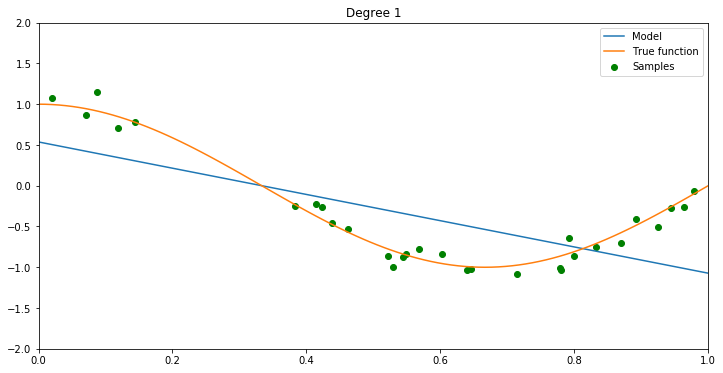### Degree 15¶

For higher degrees the model will overfit the training data, i.e. it learns the noise of the training data.

This means that we have too many parameters to be justified by the actual underlying data and therefore build an overly complex model.

The overly complex model treats fluctuations and noise as if they were intrinsic properties of the system and attempts to fit to them. The result is a model that has high variance. This means that we will not get consistent predictions of future results.

In :
make_linear_regression(15)

Score: 0.99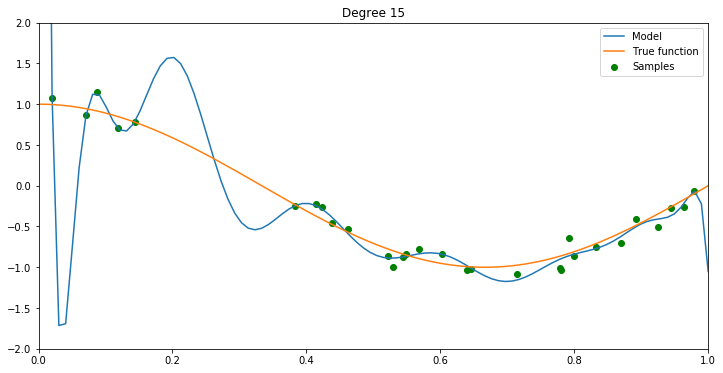### Degree 3¶

A polynomial of degree 3 approximates the true function almost perfectly, "sweet spot".

In order to find the optimal complexity we need to carefully train the model and then validate it against data that was unseen in the training set.

The performance of the model against the validation set will initially improve, but eventually suffer and dis-improve. The inflection point represents the optimal model.

In :
make_linear_regression(3)

Score: 0.97